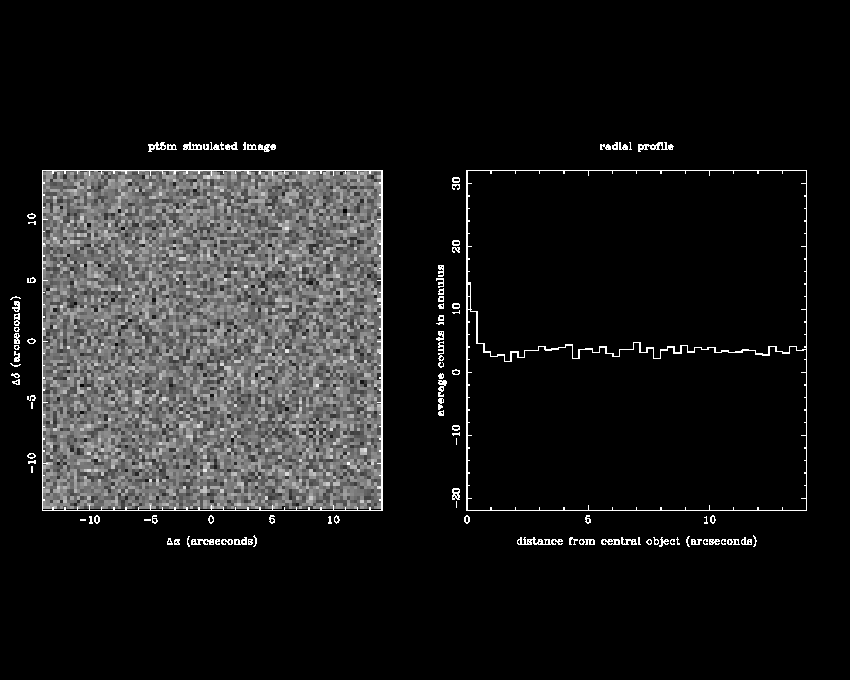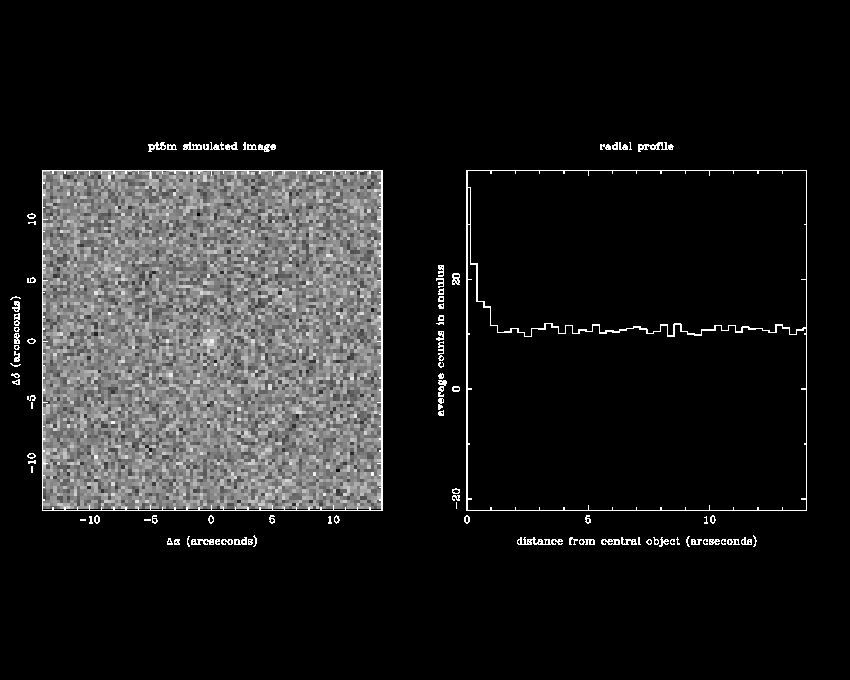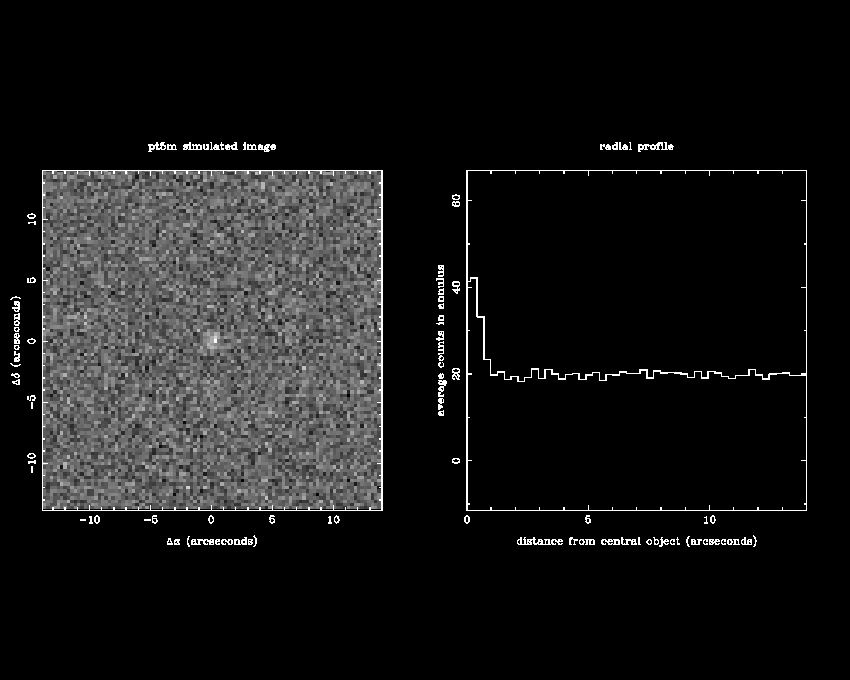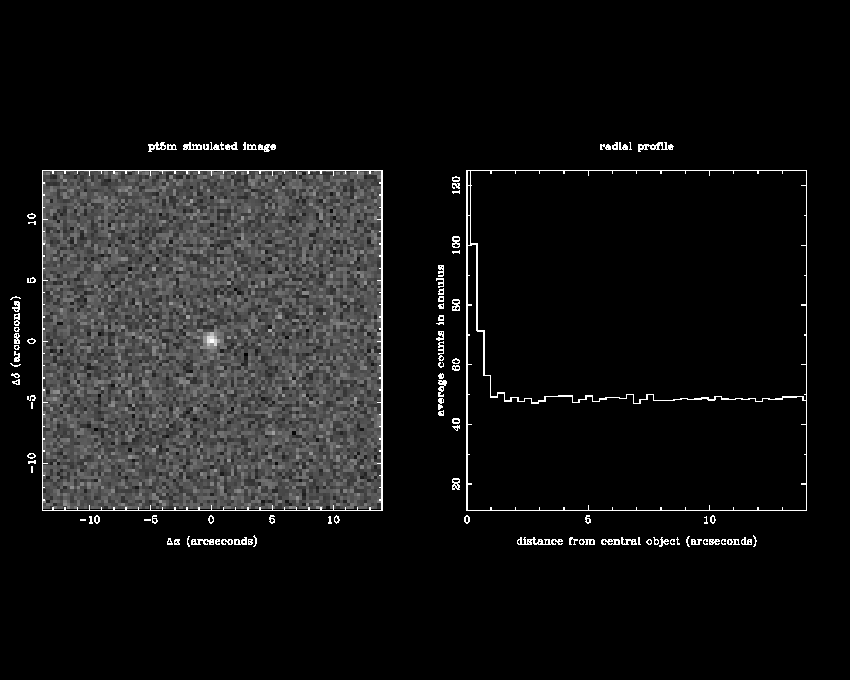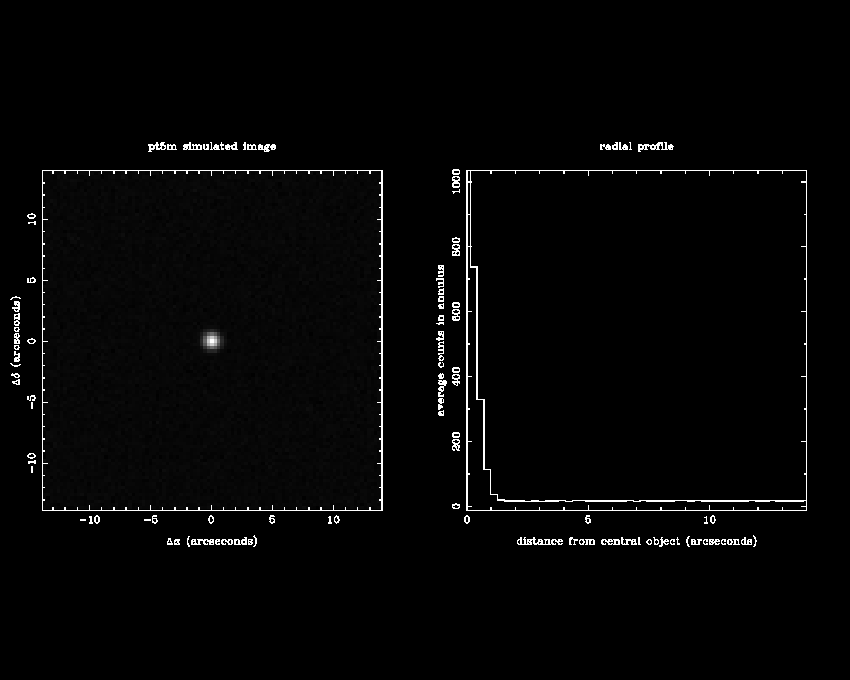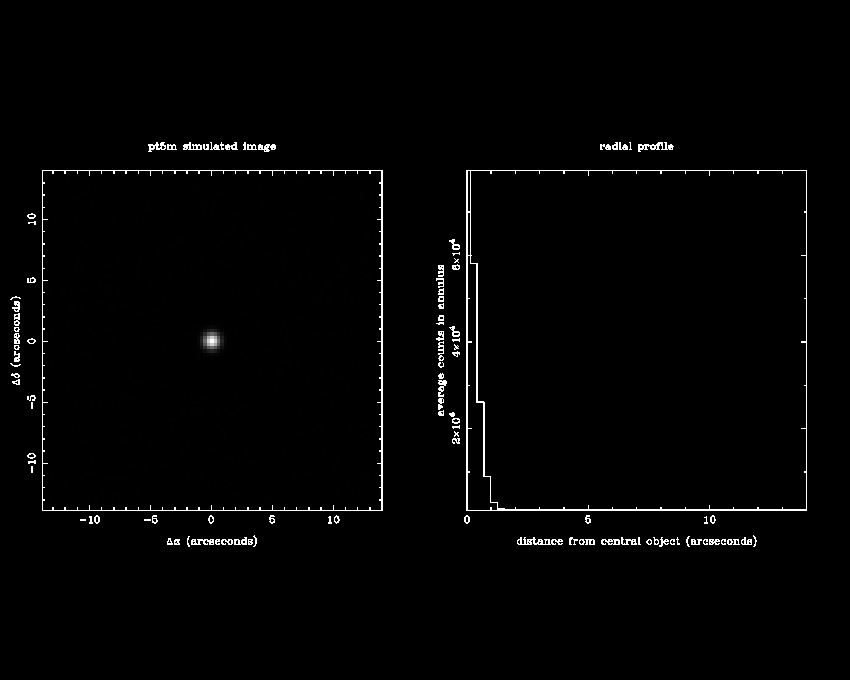Measuring the signal from an astronomical source, S, is not sufficient information on its own to determine whether or not the source is visible in an image or spectrum. A source might be bright but indistinguishable from the noise, N, if the noise is high. Conversely, a faint source might be visible if the noise is low. The correct statistic to use is therefore the signal-to-noise ratio (SNR):

SNR = signal / noise = S / N.

A signal-to-noise ratio of 10, for example, implies that the noise is one tenth of the signal. Hence the size of the error bar is 10%. The SNR required for an astronomical observation depends on the scale of the feature being studied. For example, imagine that you wish to determine the amplitude of the brightness variation of a variable star. If the amplitude of variation is, say, 0.1 magnitudes then, recalling the rule of thumb for magnitudes, this is a brightness variation of approximately 10%. It would be difficult to make out a 10% brightness variation if the typical scatter in the data (denoted by the size of the error bar) is also 10%, as demonstrated in figure 117. Hence a SNR of 10 would not be appropriate in this case.

 figure 117: Two simulated light curves of a variable star, where the amplitude of the variability is 10% of the mean signal. The top light curve has a SNR of 10, i.e. the noise, and hence the error bars, are the same size as the amplitude. The bottom light curve has a SNR of 33 and one can be much more confident that the variability has been detected.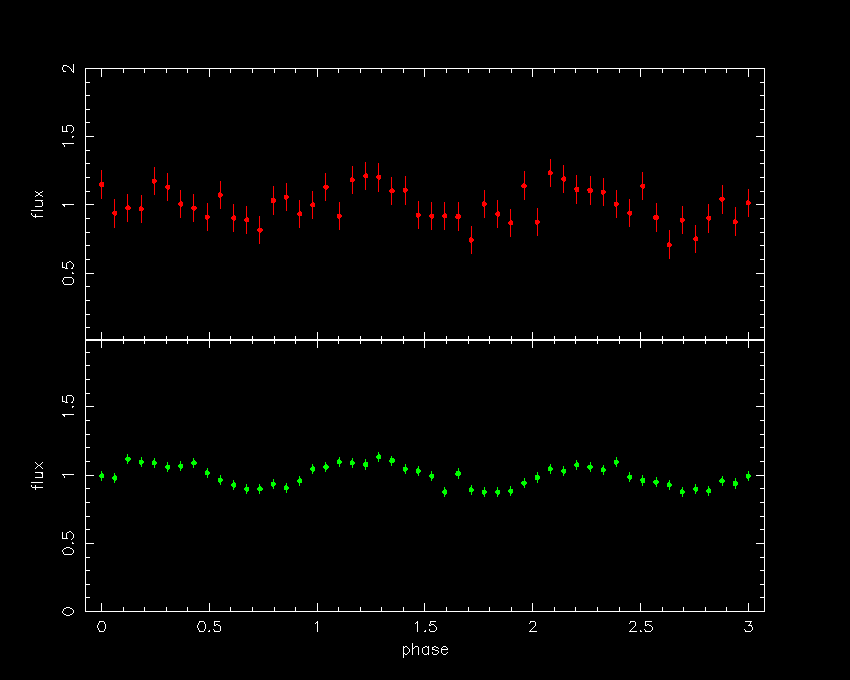So what would be an appropriate SNR? Most astronomers would not believe a result unless it is at least "three sigma", by which they mean that the quantity being measured is at least three times bigger than its error bar (denoted by the standard deviation, σ). In the above example of a variable star, the quantity we wish to measure is the amplitude of the variability, which is 10%. Hence an astronomer would not believe that a variability has been detected unless the error bar on the measured amplitude is less than 3.3%, i.e. a signal-to-noise ratio of 33 (see figure 117). Note, however, that this is a simplified and overly-conservative analysis, as the fact that there are multiple points in the light curve means that the SNR of the individual points could be worse than this and the variability would still be detectable.

Many astronomers would query even a 3σ result. Assuming that the errors on a measurement are normally distributed, one would expect a measurement to deviate by chance by 3σ from the mean once in every 370 measurements, i.e. the result is 99.7% significant. This is illustrated in figure 118. However, this assumes that there are no systematic errors in the data (e.g. in the flux calibration), which can move the position of the mean from its true value, effectively making it more likely that a 3σ result can occur by chance. To provide increased protection against systematic errors, therefore, astronomers often adopt 5σ as a measure of the believability of a result; a 5σ deviation occurs by chance once in every 1,744,278 measurements, i.e. it is 99.99994% significant.

 figure 118: Normal (or Gaussian) distribution. Regions which are less than one, two and three standard deviations (1σ, 2σ and 3σ) from the mean are coloured in different shades of blue. These regions account for 68.3%, 95.4% and 99.7% of the area under the curve, respectively.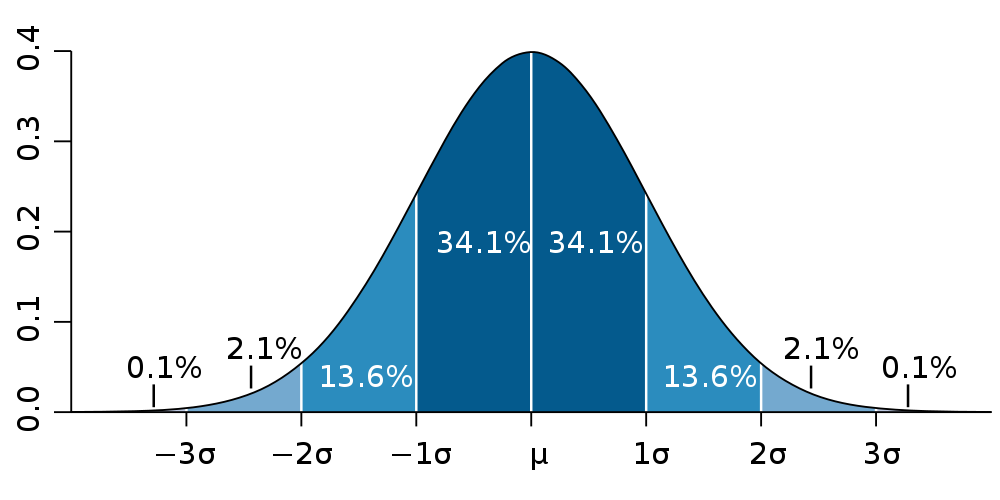It is useful to have a feel for what a stellar image of a particular SNR looks like. Simulated images of a star detected at a SNR of 1, 3, 5, 10, 100 and 1000 are shown in figure 119, along with their corresponding radial profiles (a form of cross-section through the image). As expected, the star is invisible in the SNR = 1 image, and only just visible as a faint smudge in the SNR = 3 and 5 images. The star becomes readily visible to the eye at SNR = 10, and detecting the star at SNR = 100 or higher gives good-quality data.

 figure 119: Simulated images and radial profiles of a star detected at a SNR of 1 and 3 (top panel), 5 and 10 (central panel), and 100 and 1000 (bottom panel). In each image, the maximum count level is set to white and the minimum count level to black.